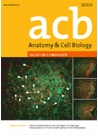상세검색
최근 검색어 전체 삭제
다국어입력
즐겨찾기0KCI등재 학술저널

# Maxillary first molar wear: a longitudinal study of children

• 등재여부 : KCI등재후보
• 2018.12
• 251 - 259 (9 pages)
• 2

The aim of this study is to examine the correlation between tooth wear and age by quantitatively measuring maxillary first molar wear in children. A total of 150 maxillary dental models were analyzed in 30 subjects (male, 11; female, 19) with an age range of 6&#8210;14 years. Maxillary first molar wear were assessed based on area, volume and the shortest distance from the buccal occlusal plane to the central pit point (BCPH). The area and volume of the tooth cusps were measured at four different offset-plane heights (0.2, 0.4, 0.6, and 0.8 mm). Relationship between age and the amount of wear or BCPH were statistically analyzed. Correlation and regression analyses were also performed, and age estimation was obtained with linear regression analysis. Repeated measures analysis of variance (ANOVA) revealed significant differences between age and the amount of wear based on area, volume, and offset-plane height. Except age of 8 and 10, 12 and 14’s 0.2-mm offset-plane-measured volume, all area and volume measurement of all ages and offset-plane height showed a significant amount of increase. Wear speeds were calculated using the BCPH. Among age and measurement variables, the correlation coefficient was strongest when the volume was measured from the 0.4-mm offset-plane. As age increases, the amount of wear, as quantified by area and volume measurements, also increases. According to this study, a regression equation that can be used for age estimation is follows: Age (y)=0.16×0.4V+0.85 (R2=0.490) using volume.

Introduction

Materials and Methods

Results

Discussion

Acknowledgements

References

로딩중